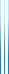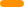# PublicationsPapers

## Tight Approximation for Unconstrained XOS Maximization

Yuval Filmus, Yasushi Kawase, Yusuke Kobayashi and Yutaro Yamaguchi
Accepted to Math of OR

We study the problem of maximizing XOS functions, which are functions that can be written as a maximum of linear functions. The number of linear functions is known as the width.

We show that the threshold inapproximability is $\Theta(n/\log n)$ in the general case, and $k-1$ for width $k$ XOS functions (where $k \geq 2$).

## BibTeX

@article{FKKY2020+,
title = {Tight Approximation for Unconstrained {XOS} Maximization},
author = {Yuval Filmus and Yasushi Kawase and Yusuke Kobayashi and Yutaro Yamaguchi},
journal = {Mathematics of Operations Research},
year = {Accepted},
}copy to clipboard